# Limit Theorems

The following article is from The Great Soviet Encyclopedia (1979). It might be outdated or ideologically biased.

## Limit Theorems

in probability theory, a group of theorems that give the conditions governing the appearance of specific regularities as a result of the action of a large number of random factors.

Historically, the first limit theorems were Bernoulli’s theorem, which was set forth in 1713, and the Laplace theorem, which was published in 1812. These theorems are concerned with the distribution of the deviations of the frequency of occurrence of an event E in n independent trials from the event’s probability p, where 0 < p < 1. The frequency is defined as the ratio m/n, where m is the number of occurrences of E in n trials. In 1837, S. Poisson extended the theorems to the case where the probability pk of the occurrence of E in the kth trial may depend on k; he described the limiting behavior, as n → ∞, of the distribution of the deviations of the frequency m/n from the arithmetic mean of the probabilities pk (1 ≤ kn):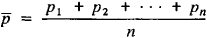If Xk denotes a random variable whose value is equal to unity when E occurs in the kth trial and is equal to zero when E does not occur, then m can be represented as the sum

m = X1 + X2 + ··· + Xn

which permits the above theorems to be treated as special cases of general limit theorems dealing with sums of independent random variables—the law of large numbers and the central limit theorem.

Law of large numbers. Suppose

(*) X1, X2, …, Xn, …

is a sequence of independent random variables and sn is the sum of the first n of the variables:

sn = X1 + X2 + · · · + Xn

Let An and Bn2 be respectively the mathematical expectation

An = E sn = EX1 + EX2 + · · · + EXn

and the variance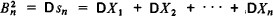of the sum sn. The sequence (*) is said to obey the law of large numbers if for any ∊ > 0 the probability of the inequality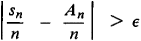approaches zero as n → ∞.

Broad conditions for the applicability of the law of large numbers were first obtained by P. L. Chebyshev in 1867. The conditions were subsequently generalized by A. A. Markov (the elder). The problem of the necessary and sufficient conditions for the applicability of the law of large numbers was definitively solved by A. N. Kolmogorov in 1928. When the Xn have a common distribution function, these conditions, as shown by A. Ia. Khinchin in 1929, reduce to a single condition: the Xn must have finite mathematical expectations.

Central limit theorem. The central limit theorem is applicable to the sequence (*) if for any z1 and z2 the limit of the probability of the inequality

Z1Bn < sn - An < z2Bn

as n → ∞ is the quantity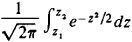Fairly general sufficient conditions for the applicability of the central limit theorem were given by Chebyshev in 1887. Gaps, however, were found in his proof that were filled only later by Markov, in 1898. An almost definitive solution of the problem was obtained by A. M. Liapunov in 1901. A precise formulation of the Liapunov theorem is as follows. Let

ck = E ǀXk – EXkǀ 2+δ δ > 0

Cn = c1 + c2 + ··· + cn

If the ratio Ln = Cn/Bn2+δ approaches zero as n → ∞, then the central limit theorem is applicable to the sequence (*). A definite solution of the problem of the conditions of applicability of the central limit theorem was obtained in its general outlines by S. N. Bernshtein in 1926 and completed by W. Feller in 1935.

Other trends in the study of limit theorems include the following.

(1) Investigations were begun by Markov and continued by Bernshtein and others on the conditions governing the applicability of the law of large numbers and the central limit theorem to sums of dependent variables.

(2) Even in the case of a sequence of identically distributed random variables simple examples can be given where the sums have a limiting distribution different from a normal distribution (nondegenerate distributions are meant here—that is, distributions that are not concentrated entirely at one point). In the papers of such mathematicians as the Soviets A. Ia. Khinchin and B. V. Gnedenko and the Frenchmen P. Lévy and W. Dublin, a complete study has been made of both the class of possible limiting distributions for sums of independent random variables and the conditions for convergence of the distributions of the sums to specific limiting distributions.

(3) Considerable attention has been devoted to local limit theorems. For example, let the variables Xn take on only integral values. Then the sums sn also assume only integral values, and the question naturally arises of the limiting behavior of the probabilities Pn(m) that sn = m, where m is an integer. A simple example of a local limit theorem is the Laplace theorem.

(4) Limit theorems in their classical formulation describe the behavior of individual sums sn as n increases. Sufficiently general limit theorems for the probabilities of events that depend simultaneously on several sums were obtained for the first time by Kolmogorov in 1931. It follows from his results, for example, that under very broad conditions the limit of the probability of the inequalityis the quantity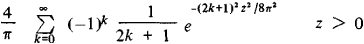(5) The above limit theorems are concerned with sums of random variables. Limit theorems of a different kind are exemplified by limit theorems for the terms of an ordered series. Such theorems have been studied in detail by the Soviet mathematicians B. V. Gnedenko and N. V. Smirnov.

(6) Finally, also classed with limit theorems are theorems that establish for sequences of random variables properties occurring with a probability equal to unity. An example is the law of iterated logarithm.

### REFERENCES

Gnedenko, B. V., and A. N. Kolmogorov. Predel’nye raspredeleniia dlia summ nezavisimykh sluchainykh velichin. Moscow-Leningrad, 1949.
Ibragimov, I. A., and Iu. V. Linnik. Nezavisimye i statsionarno sviazannye velichiny. Moscow, 1965.
Prokhorov, Iu. V., and Iu. A. Rozanov. Teoriia veroiatnostei: Osnovnye poniatiia: Predel’nye teoremy: Sluchainye protsessy, 2nd ed. Moscow, 1973.

IU. V. PROKHOROV

References in periodicals archive ?
It then moves on to cover the Lebesgue measures, integration on the real line, basic limit theorems, and normed vector spaces.
For the proof of above theorems, we apply a probabilistic approach based on limit theorems for weakly convergent probability measures in the space H(D).
Among others, we can cite strong approximation results [8-10], laws of the iterated logarithm [11-13], limit theorems for correlated sceneries or walks [14-17], large and moderate deviations results [18-22], and ergodic and mixing properties (see the survey ).
Quantum central limit theorems are quantum analogs of the classical central limit theorem, which deal with observables from a quantum probability point of view.
The second contribution is an account of limit theorems for the DMBP when S is not positive recurrent.
We also show that this approach can be used to obtain strong limit theorems in cases where previously only distributional convergence was known.
Moreover, for the negatively associated sequence, Newman  established the central limit theorem, Matula  studied the three series theorems; Shao and Su  obtained the law of the iterated logarithm; Jing and Liang  gave the strong limit theorems for weighted sums.
Appendices present standard probability distributions, proofs of limit theorems, and programming information for R and Stan.
Guyon  has given the definition of bifurcating Markov chains indexed by binary tree and studied their limit theorems. Berger and Ye  have studied the existence of entropy rate for some stationary random fields on a homogeneous tree.
Newman, "Asymptotic independence and limit theorems for positively and negatively dependent random variables," in Inequalities in Statistics and Probability, Y.
Rosenbaum, Central limit theorems for realized volatility under hitting times of an irregular grid, Stochastic Process.

Site: Follow: Share:
Open / Close